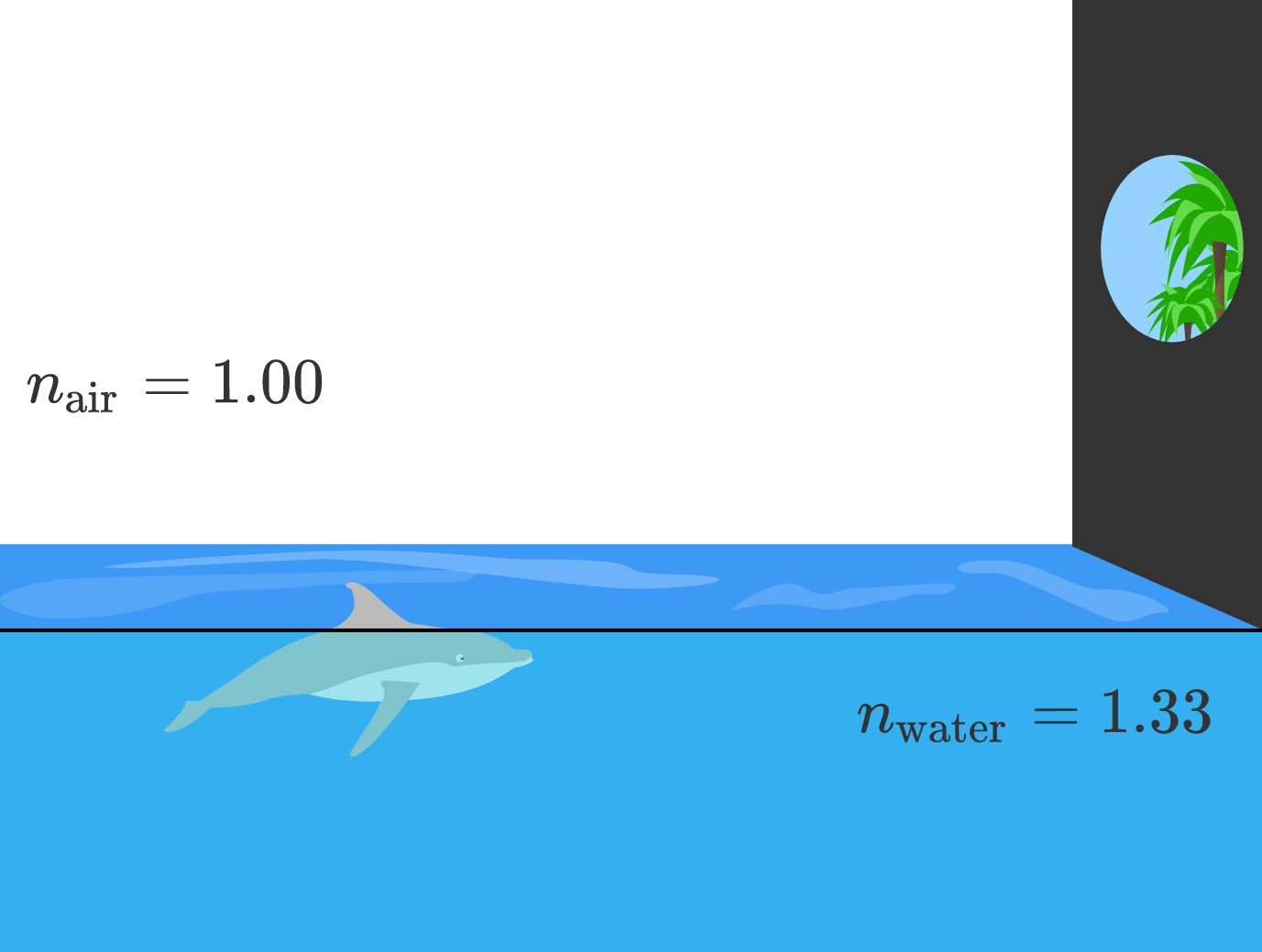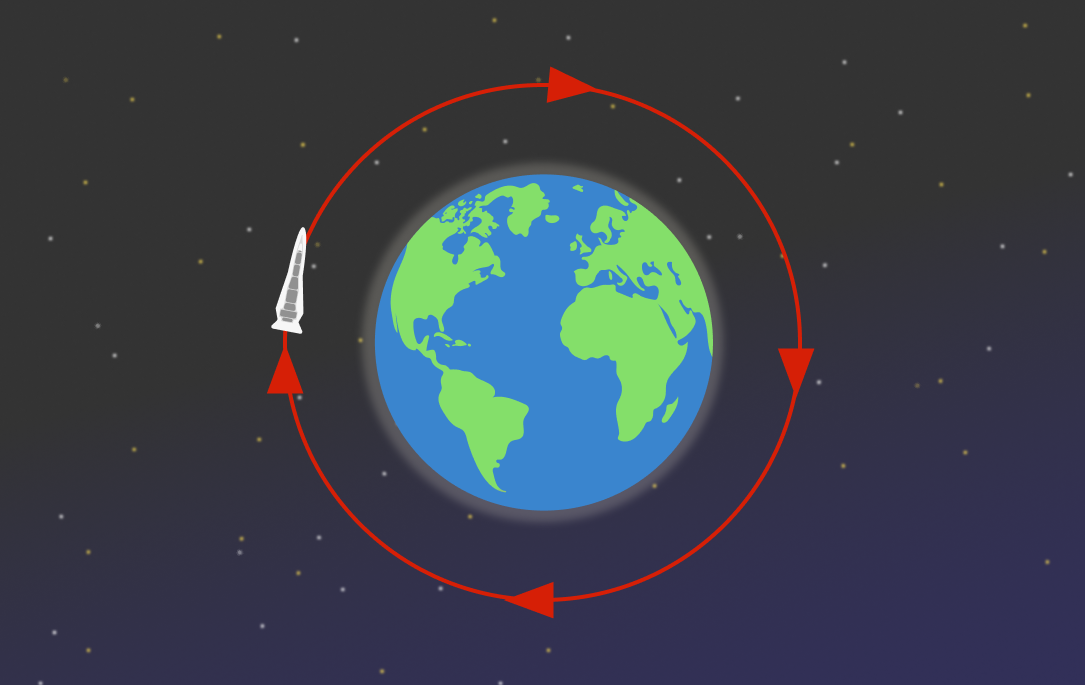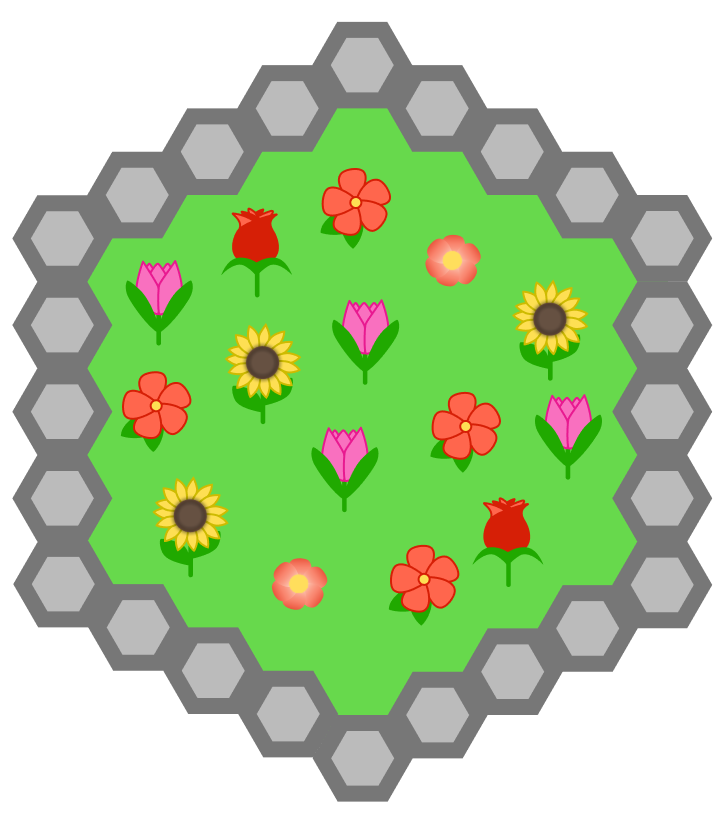# Problems of the Week

Contribute a problem

# 2018-07-16 Intermediate

Ecco, a dolphin, is trying to jump through a circular hole in his enclosure to escape from the aquarium.

From Ecco's perspective just under the water's surface, does the hole seem easier or harder to fit through? And does it seem higher or lower?Select one or more

How fast $($in $\text{m/s})$ does a spaceship need to orbit around Earth to not spiral down or fly away from it?Details and Assumptions:

• The spaceship is $\SI{400}{\kilo\meter}$ above the surface of Earth.
• Earth's radius is $\SI{6371}{\kilo\meter}.$
• Gravitational acceleration on the spaceship is $\SI[per-mode=symbol]{8.69}{\meter\per\second\squared}$ towards the center of Earth.The diagonals of a regular pentagon can be used to form a smaller regular pentagon.

The ratio of the areas of the large pentagon to the small pentagon is $\phi^n$, where $\phi$ is the golden ratio $\frac{1 + \sqrt{5}}{2}$, and $n$ is an integer.

What is $n?$

A monic polynomial $f(x)$ of degree 5 is such that $\begin{cases} f(0)=1 \\ f(1)=2 \\ f(2)=5 \\ f(3)=10 \\ f(4)=17. \end{cases}$ What is the value of $f(5)?$

Regular hexagon patio blocks of area 1 are used to outline the garden shown, with $n=5$ blocks on each side placed edge to edge.

If $n=202,$ then what is the area of the garden enclosed by the path?×